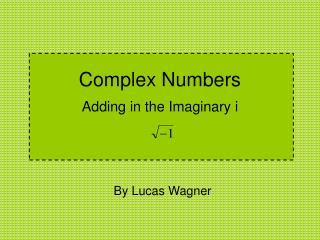Download PresentationComplex Numbers

# Complex Numbers - PowerPoint PPT PresentationDownload Presentation## Complex Numbers

- - - - - - - - - - - - - - - - - - - - - - - - - - - E N D - - - - - - - - - - - - - - - - - - - - - - - - - - -
##### Presentation Transcript

1. Complex Numbers Adding in the Imaginary i By Lucas Wagner

2. The Domain of the Square Root We might think of as a parabola on its side, with the following equivalent statement: So we can see that negative values of x do not yield any real y-values.

3. The Quadratic Formula In a mathematically thorough and rigorous manner we can apply the quadratic formula, to any equation that can put in this form: But we can see the possibility of problems occurring: there is no mathematical requirement that the number under the radical, , be positive, as the following example shows.

4. Girolamo’s Problem In The Great Art, published in 1545, Girolamo Cardano discusses the following problem. No Intersection! To find x and y, use substitution. Apply the Quadratic Formula. Due to the symmetry in the problem, x and y take on ± values.

5. Bombelli works with Imaginary Numbers Rafael Bombelli in the 1560’s figured out a way to work with imaginary numbers. We write this in modern notation as: Using these rules, Bombelli worked Cardano’s cubic solutions (Sketch 11 HW) to arrive at real results, so he wasn’t just interested in imaginary numbers for themselves.

6. Fundamental Theorem of Algebra Any polynomial of degree n,with n greater than zero (a non-constant polynomial), has n roots. In other words, pn(x) = 0 has n solutions. • René Descartes and Albert Girard in the 1600s had their suspicions that this was the case, if they allowed for three different kinds of roots: • Positive (considered “real”) • Negative (considered “false” at the time) • Imaginary (Complex numbers) Various mathematicians have tried their hand at proving this theorem: Leonhard Euler (1749), Pierre-Simon Laplace (1795), and Carl Friedrich Gauss (1799), to name a few. Wikipedia – Fundamental Theorem of Algebra

7. Real and Imaginary Parts of Complex Numbers When working with complex numbers, it is shown that breaking the number into the sum of a real and imaginary part maintains a good algebraic field. For example, Using FOIL and Bombelli’s rules, we can find the product of z and w.

8. Complex Numbers as Vectors Jean-Robert Argand in 1806 came up with the idea of a geometrical interpretation of complex numbers. Replace the x-axis with the real part of complex numbers, and the y-axis with the imaginary part. Thus, has the graphical interpretation,

9. Trigonometric Formulas and Complex Numbers One can derive Euler’s formula from the Taylor series representations of sine, cosine, and the exponential, and the rules developed by Bombelli. The result is the following: One can derive many familiar trigonometric formulas using Euler’s formula and the properties of the exponent, i.e.: These formulas can be used to derive Abraham De Moivre’s formula (though in history De Moivre’s formula came before Euler’s):

10. Polar Form of Vectors The complex number z also has a polar form. It uses Euler’s formula as its backbone. The r gives the length of the vector, and eiθgives the direction.

11. Physics Application:Centripetal Motion Consider an object moving in a circle of radius r with an angular frequency of ω. What is its velocity and acceleration? Create a parametrization of the position in the complex plane, Assuming that we can take derivatives like usual, Multiplication of complex numbers is a rotation in the complex plane. In this case, the ieiωtgives us the direction tangent to the circle. The direction of the acceleration here is –eiωt, which is in towards the center of the circle. Thus we can establish the following: